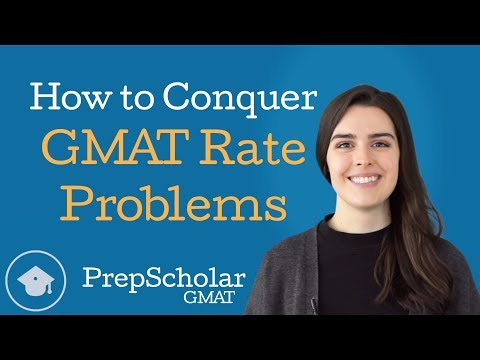How to Conquer GMAT Rate Problems:

by on January 30th, 2018GMAT rate problems are a constant on the Quantitative section. Many students dread rate problems because they aren’t quick or easy—they often require a series of steps to get to the answer. To make matters worse, this series of steps is usually not immediately obvious because there are so many elements to the problem and because these elements are usually presented as part of a difficult-to-interpret word problem scenario. This means that seeing a clear path to the finish line is difficult with many GMAT rate problems.

So what should we do when we run into a rate problem that we just can’t see how to solve? In “How to Conquer GMAT Rate Problems”, we discuss one of the most valuable GMAT math strategies—taking complex problems step-by-step.

GMAT rate problems give us limited information that we can use in limited ways. At any given step, we are only able to do one or two things with the information we have. Because our options at each step are so limited, we can usually make our way to the answer by figuring out only the next step we are able to take now given the information we have, then repeating the process with the information we learn at each step until we reach our answer. While working out a full, multi-step game plan up top can be overwhelming, simply answering “What can I do with this information?” at each step is more manageable and will eventually lead us to our answer.

In the case of GMAT rate problems, the next step we are able to take is usually doing the next possible conversion—converting the value we have into any another value we can using rates from the problem. We walk through exactly what this means by solving one of the real GMAT rate problems from the GMATPrep software.

Watch the video to see how it’s done!

With this strategy, you’re ready for any multi-step rate problems GMAT test writers can throw at you. However, this isn’t all—taking problems one step at a time when the solution isn’t immediately clear is one of the broader GMAT math strategies, as we can apply it to a variety of multi-step problems. Not sure what to do with a bunch of terms with exponents? See how you can combine two or three of them, then see if it simplifies further with your new term. Have a complicated geometric figure? See which angle or side you can solve for now, then see if you can solve for more with the new information.

As a final note, this strategy applies best to distance rate problems, but it can also be used on certain work rate and interest rate questions. If you’d like more detailed information on how to solve each of these specific types of rate problem, check out our rundown of the three types of GMAT rate problems.

To stay updated on our latest GMAT videos, you can subscribe to our new PrepScholar GMAT YouTube channel—we’ll have three new free videos every month.

Happy GMAT studies!

The post How to Conquer GMAT Rate Problems appeared first on Online GMAT Prep Blog by PrepScholar.